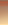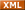# 李sir_Blog博客园 首页 联系 订阅管理
OpenMP是一个支持共享存储并行设计的库，特别适宜多核CPU上的并行程序设计。今天在双核CPU机器上试了一下OpenMP并行程序设计，发现效率方面超出想象，因此写出来分享给大家。

int main(int argc, char* argv[])
{
#pragma omp parallel for
for (int i = 0; i < 10; i++ )
{
printf("i = %d/n", i);
}
return 0;
}

i = 0
i = 5
i = 1
i = 6
i = 2
i = 7
i = 3
i = 8
i = 4
i = 9

将for循环里的语句变成并行执行后效率会不会提高呢，我想这是我们最关心的内容了。下面就写一个简单的测试程序来测试一下：

void test()
{
int a = 0;
clock_t t1 = clock();
for (int i = 0; i < 100000000; i++)
{
a = i+1;
}
clock_t t2 = clock();
printf("Time = %d/n", t2-t1);
}

int main(int argc, char* argv[])
{
clock_t t1 = clock();
#pragma omp parallel for
for ( int j = 0; j < 2; j++ ){
test();
}
clock_t t2 = clock();
printf("Total time = %d/n", t2-t1);

test();
return 0;
}

Time = 297
Time = 297
Total time = 297
Time = 297

1、fork/join并行执行模式的概念
OpenMP是一个编译器指令和库函数的集合，主要是为共享式存储计算机上的并行程序设计使用的。

int main(int argc, char* argv[])
{
clock_t t1 = clock();
#pragma omp parallel for
for ( int j = 0; j < 2; j++ ){
test();
}
clock_t t2 = clock();
printf("Total time = %d/n", t2-t1);

test();
return 0;
}

2、OpenMP指令和库函数介绍

pragma omp 指令 [子句[子句]…]

#pragma omp parallel private(i, j)
parallel 就是指令， private是子句

OpenMP的指令有以下一些：
parallel，用在一个代码段之前，表示这段代码将被多个线程并行执行
for，用于for循环之前，将循环分配到多个线程中并行执行，必须保证每次循环之间无相关性。
parallel for， parallel 和 for语句的结合，也是用在一个for循环之前，表示for循环的代码将被多个线程并行执行。
sections，用在可能会被并行执行的代码段之前
parallel sections，parallel和sections两个语句的结合
critical，用在一段代码临界区之前
single，用在一段只被单个线程执行的代码段之前，表示后面的代码段将被单线程执行。
flush
barrier，用于并行区内代码的线程同步，所有线程执行到barrier时要停止，直到所有线程都执行到barrier时才继续往下执行。
atomic，用于指定一块内存区域被制动更新
master，用于指定一段代码块由主线程执行
ordered， 用于指定并行区域的循环按顺序执行
OpenMP除上述指令外，还有一些库函数，下面列出几个常用的库函数：
omp_get_num_procs, 返回运行本线程的多处理机的处理器个数。
omp_init_lock, 初始化一个简单锁
omp_set_lock， 上锁操作
omp_unset_lock， 解锁操作，要和omp_set_lock函数配对使用。
omp_destroy_lock， omp_init_lock函数的配对操作函数，关闭一个锁

OpenMP的子句有以下一些
private, 指定每个线程都有它自己的变量私有副本。
firstprivate指定每个线程都有它自己的变量私有副本，并且变量要被继承主线程中的初值。
lastprivate主要是用来指定将线程中的私有变量的值在并行处理结束后复制回主线程中的对应变量。
reduce用来指定一个或多个变量是私有的，并且在并行处理结束后这些变量要执行指定的运算。
nowait忽略指定中暗含的等待
schedule指定如何调度for循环迭代
shared指定一个或多个变量为多个线程间的共享变量
ordered用来指定for循环的执行要按顺序执行
copyprivate用于single指令中的指定变量为多个线程的共享变量
default用来指定并行处理区域内的变量的使用方式，缺省是shared
3、parallel 指令的用法
parallel 是用来构造一个并行块的，也可以使用其他指令如for、sections等和它配合使用。

#pragma omp parallel [for | sections] [子句[子句]…]
{
//代码
}
parallel语句后面要跟一个大括号对将要并行执行的代码括起来。
void main(int argc, char *argv[]) {
#pragma omp parallel
{
printf(“Hello, World!/n”);
}
}

Hello, World!
Hello, World!
Hello, World!
Hello, World!

void main(int argc, char *argv[]) {
{
}
}

4、for指令的使用方法
for指令则是用来将一个for循环分配到多个线程中执行。for指令一般可以和parallel指令合起来形成parallel for指令使用，也可以单独用在parallel语句的并行块中。
#pragma omp [parallel] for [子句]
for循环语句

int j = 0;
#pragma omp for
for ( j = 0; j < 4; j++ ){
}

j = 0, ThreadId = 0
j = 1, ThreadId = 0
j = 2, ThreadId = 0
j = 3, ThreadId = 0

int j = 0;
#pragma omp parallel for
for ( j = 0; j < 4; j++ ){
}

j = 0, ThreadId = 0
j = 2, ThreadId = 2
j = 1, ThreadId = 1
j = 3, ThreadId = 3

int j = 0;
#pragma omp parallel
{
#pragma omp for
for ( j = 0; j < 4; j++ ){
}
}

j = 1, ThreadId = 1
j = 3, ThreadId = 3
j = 2, ThreadId = 2
j = 0, ThreadId = 0

int j;
#pragma omp parallel
{
#pragma omp for
for ( j = 0; j < 100; j++ ){

}
#pragma omp for
for (  j = 0; j < 100; j++ ){

}
}

for 循环语句中，书写是需要按照一定规范来写才可以的，即for循环小括号内的语句要按照一定的规范进行书写，for语句小括号里共有三条语句
for( i=start; i < end; i++)

i=start; 是for循环里的第一条语句，必须写成 “变量＝初值” 的方式。如 i＝0
i < end;是for循环里的第二条语句，这个语句里可以写成以下4种形式之一：

i++
++i
i--
--i
i += inc
i -= inc
i = i + inc
i = inc + i
i = i –inc

5 sections和section指令的用法
section语句是用在sections语句里用来将sections语句里的代码划分成几个不同的段，每段都并行执行。用法如下：
#pragma omp [parallel] sections [子句]
{
#pragma omp section
{
代码块
}
}

void main(int argc, char *argv)
{
#pragma omp parallel sections {
#pragma omp section
#pragma omp section
#pragma omp section
#pragma omp section
}

void main(int argc, char *argv)
{
#pragma omp parallel {
#pragma omp sections
{
#pragma omp section
#pragma omp section
}
#pragma omp sections
{

#pragma omp section
#pragma omp section
}
}